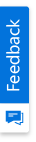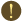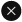We use cookies to give you the best experience on our website. If you continue to browse, then you agree to our privacy policy and cookie policy.Unfortunately, activation email could not send to your email. Please try again.# How to render a line at a specific axis value in Cartesian charts (SfCartesianChart) ?

Platform: Flutter |
Control: SfCartesianChart

In this article we explain how to render a line at the specific value using the Flutter SfCartesianChart widget.

Our Flutter Cartesian chart provides an option to render a line at a specific value using the plot band feature. The following steps explains how to add plot band feature in both x and y axes to add a line.

### Render a line in x-axis

Step 1: Declare and initialize the required data source for the chart.

```final List<ChartSampleData> chartData = <ChartSampleData>[
ChartSampleData(x: 'Jan', y: 23),
ChartSampleData(x: 'Feb', y: 24),
ChartSampleData(x: 'Mar', y: 23),
ChartSampleData(x: 'Apr', y: 22),
ChartSampleData(x: 'May', y: 21),
ChartSampleData(x: 'Jun', y: 27),
ChartSampleData(x: 'Jul', y: 33),
ChartSampleData(x: 'Aug', y: 36),
ChartSampleData(x: 'Sep', y: 23),
ChartSampleData(x: 'Oct', y: 25),
ChartSampleData(x: 'Nov', y: 22)
];
```

Step 2: Then initialize the SfCartesianChart widget and then initialize the axes with other required properties.

```SfCartesianChart(
primaryXAxis: CategoryAxis(),
primaryYAxis: NumericAxis(),
series: <ChartSeries<ChartSampleData, String>>[
LineSeries<ChartSampleData, String>(
dataSource: chartData,
xValueMapper: (ChartSampleData data, _) => data.x,
yValueMapper: (ChartSampleData data, _) => data.y)
],
)

```

Step 3: Then add the plotBands property to the primaryXAxis and set the start and end value of the plot band as same value.

Here we have used the category axis as primaryXAxis, so you need to specify the index value in the start and end properties.

```SfCartesianChart(
primaryXAxis: CategoryAxis(
plotBands: <PlotBand>[
PlotBand(
start: 7,
end: 7,
//Specify the width for the line
borderWidth: 2,
//Specify the dash array for the line
dashArray: const <double>[4, 5])
]),
primaryYAxis: NumericAxis(),
series: <ChartSeries<ChartSampleData, String>>[
LineSeries<ChartSampleData, String>(
dataSource: chartData,
xValueMapper: (ChartSampleData data, _) => data.x,
yValueMapper: (ChartSampleData data, _) => data.y)
],
)
```### Render a line in y-axis

Step 1: Declare and initialize the required data source for the chart.

```final List<ChartSampleData> chartData = <ChartSampleData>[
ChartSampleData(x: 'Jan', y: 23),
ChartSampleData(x: 'Feb', y: 24),
ChartSampleData(x: 'Mar', y: 23),
ChartSampleData(x: 'Apr', y: 22),
ChartSampleData(x: 'May', y: 21),
ChartSampleData(x: 'Jun', y: 27),
ChartSampleData(x: 'Jul', y: 33),
ChartSampleData(x: 'Aug', y: 36),
ChartSampleData(x: 'Sep', y: 23),
ChartSampleData(x: 'Oct', y: 25),
ChartSampleData(x: 'Nov', y: 22)
];
```

Step 2: Then initialize the SfCartesianChart widget and then initialize the axes with other required properties.

```SfCartesianChart(
primaryXAxis: CategoryAxis(),
primaryYAxis: NumericAxis(),
series: <ChartSeries<ChartSampleData, String>>[
LineSeries<ChartSampleData, String>(
dataSource: chartData,
xValueMapper: (ChartSampleData data, _) => data.x,
yValueMapper: (ChartSampleData data, _) => data.y)
],
)

```

Step 3: Then add the plotBands property to the primaryYAxis and set the start and end value of the plot band as same value.

```SfCartesianChart(
primaryXAxis: CategoryAxis(),
primaryYAxis: NumericAxis(
plotBands: <PlotBand>[
PlotBand(
start: 36,
end: 36,
//Specify the width for the line
borderWidth: 2,
//Specify the dash array for the line
dashArray: const <double>[4, 5])
]),
series: <ChartSeries<ChartSampleData, String>> [
LineSeries<ChartSampleData, String> (
dataSource: chartData,
xValueMapper: (ChartSampleData data, _) => data.x,
yValueMapper: (ChartSampleData data, _) => data.y)
],
)
```View the sample in GitHubYou are using an outdated version of Internet Explorer that may not display all features of this and other websites. Upgrade to Internet Explorer 8 or newer for a better experience.